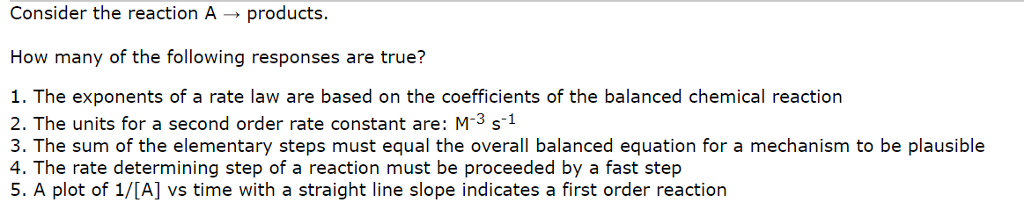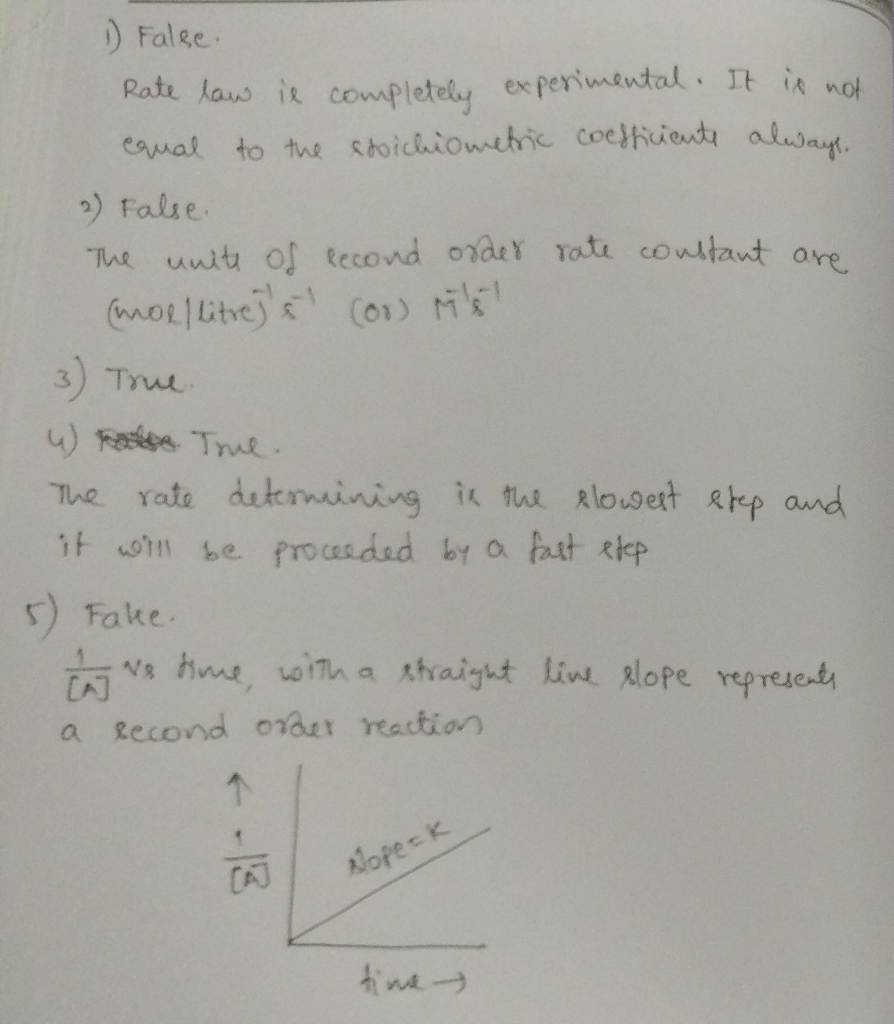# Question & Answer: Consider the reaction A rightarrow products. How many of the following responses are true?…..

Explain pleaseConsider the reaction A rightarrow products. How many of the following responses are true? The exponents of a rate law are based on the coefficients of the balanced chemical reaction The units for a second order rate constant are: M^-3 s^-1 The sum of the elementary steps must equal the overall balanced equation for a mechanism to be plausible The rate determining step of a reaction must be proceeded by a fast step A plot of 1/[A] vs time with a straight line slope indicates a first order reaction